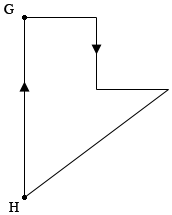SEARCH HOMEMath Central Quandaries & QueriesQuestion from Ruby: Amy drove on the highways at 70 miles/hour to pick up fruits from her grandma’s garden in the morning. She stayed there for two hours. When coming back in the afternoon, she drove on the local roads at 40 miles per hour to avoid traffic. She spent a total of 8 hours for this trip, and drove a total 300 miles. How many miles did she drive each way? I need a visual representations to show thinking process and to solve the problemHi Ruby,

Here is my diagram of Amy's trip. She drove from her home $H$ to grandma's house $H$ on a direct fast route. In the afternoon she drove home on local roads, a more indirect route.Suppose the distance she travelled to grandma's house was $d_1$ miles and it took her $t_1$ hours, and the distance she travelled from grandma's house to home was $d_2$ miles and it took her $t_2$ hours.

What facts do you know? The total distance she travelled was 300 miles so

$d_1 + d_2 = 300 \mbox{ miles.}$

She spent $t_1 + t_2$ hours driving and 2 hours at grandma's house and her total time was 8 hours. Thus

$t_1 + t_2 + 2 = 8 \mbox{ hours.}$

On the trip to grandma's house she drove $d_1$ miles in $t_1$ hours and her speed was 70 miles per hour so

$70 = \frac{d_1}{t_1} \mbox{ or } 70 \times t_1 = d_{1}.$

Likewise for the return trip we get

$40 \times t_2 = d_{2}.$

I would suggest that you solve the first equation for $d_1$ and the second equation for $t_1$ and substitute these values into the third equation. This converts the third equation to one involving $d_2$ and $t_{2}.$ The fourth equation also involves $d_2$ and $t_{2}.$ Solve these two equations for $d_2$ and $t_{2}.$

PennyMath Central is supported by the University of Regina and The Pacific Institute for the Mathematical Sciences.